# Topics - LED Strip Effects - Two fire effects

Page 1 of 1

## LED Strip Effects – Two fire effectsWelcome to the Tweaking4All community forums!
When participating, please keep the Forum Rules in mind!

Topics for particular software or systems: Start your topic link with the name of the application or system.
Examples: "MacOS X - Your question", "MS Word - Your Tip or Trick".

Please note that switching to another language when reading a post will not work!
Posts will not have a translated counterpart.

Home Forums Hardware Arduino LED Strip Effects – Two fire effects

Viewing 10 posts - 1 through 10 (of 10 total)
• Author
Posts
• 6969

This is the continuation of a comment posted by Steve:

Hi Guys
Looking for major help, I have made an intinity mirror which is using 205 ws2812B what I would like to do is use the fire sketch (which i can of course) but what i cannot do or maybe its not possiable is to have 2 starting point with the flames using 100 leds.
starting point (1) leds 1 to 100
starting point (2) leds 205 to 105
The idea is that it looks like the flames are starting at the same point and lapping round the mirror
I really enjoy working with the arduino and leds but sometimes its so bloody frustating :)
If you think its not possiable let me know as i will then give up on that idea, on a postive note if it is any help would be much appreciated
Fantastic page by the way
6971

I wouldn’t think of it as impossible, would it be OK if both sets behave the same with the fire effect? Or should they be different?

6973

OK, let me post the original Fire Effect here:

```void loop() {  Fire(55,120,15);}
void Fire(int Cooling, int Sparking, int SpeedDelay) {  static byte heat[NUM_LEDS];  int cooldown;    // Step 1.  Cool down every cell a little  for( int i = 0; i < NUM_LEDS; i++) {    cooldown = random(0, ((Cooling * 10) / NUM_LEDS) + 2);        if(cooldown>heat[i]) {      heat[i]=0;    } else {      heat[i]=heat[i]-cooldown;    }  }    // Step 2.  Heat from each cell drifts 'up' and diffuses a little  for( int k= NUM_LEDS - 1; k >= 2; k--) {    heat[k] = (heat[k - 1] + heat[k - 2] + heat[k - 2]) / 3;  }      // Step 3.  Randomly ignite new 'sparks' near the bottom  if( random(255) < Sparking ) {    int y = random(7);    heat[y] = heat[y] + random(160,255);    //heat[y] = random(160,255);  }  // Step 4.  Convert heat to LED colors  for( int j = 0; j < NUM_LEDS; j++) {    setPixelHeatColor(j, heat[j] );  }  showStrip();  delay(SpeedDelay);}void setPixelHeatColor (int Pixel, byte temperature) {  // Scale 'heat' down from 0-255 to 0-191  byte t192 = round((temperature/255.0)*191);   // calculate ramp up from  byte heatramp = t192 & 0x3F; // 0..63  heatramp <<= 2; // scale up to 0..252   // figure out which third of the spectrum we're in:  if( t192 > 0x80) {                     // hottest    setPixel(Pixel, 255, 255, heatramp);  } else if( t192 > 0x40 ) {             // middle    setPixel(Pixel, 255, heatramp, 0);  } else {                               // coolest    setPixel(Pixel, heatramp, 0, 0);  }}```
6975

Sine you want two sets:

Set 1: leds 1 – 100
Set 2: leds 105 – 205

We could “cheat” a little with minimal modifications.
In “Step 4” we set the LED color, for each LED. So we could cheat by copying the color from set1 to set2:

`for( int j = 0; j < NUM_LEDS; j++) {    setPixelHeatColor(j, heat[j] );    setPixelHeatColor(j + 104, heat[j] );  }`

Now this will work but we’d run into trouble with the use of NUM_LEDS, so we define a new define for that.

`#define SET_NUM_LEDS 100`

and modify the previous code as such:

```#define SET_NUM_LEDS 100  // might need to tweak this number
...
void loop() {  Fire(55,120,15);}
void Fire(int Cooling, int Sparking, int SpeedDelay) {  static byte heat[SET_NUM_LEDS];  int cooldown;    // Step 1.  Cool down every cell a little  for( int i = 0; i < SET_NUM_LEDS; i++) {    cooldown = random(0, ((Cooling * 10) / SET_NUM_LEDS) + 2);        if(cooldown>heat[i]) {      heat[i]=0;    } else {      heat[i]=heat[i]-cooldown;    }  }    // Step 2.  Heat from each cell drifts 'up' and diffuses a little  for( int k= SET_NUM_LEDS - 1; k >= 2; k--) {    heat[k] = (heat[k - 1] + heat[k - 2] + heat[k - 2]) / 3;  }      // Step 3.  Randomly ignite new 'sparks' near the bottom  if( random(255) < Sparking ) {    int y = random(7);    heat[y] = heat[y] + random(160,255);    //heat[y] = random(160,255);  }  // Step 4.  Convert heat to LED colors  for( int j = 0; j < SET_NUM_LEDS; j++) {    setPixelHeatColor(j, heat[j] );    setPixelHeatColor(j + 105, heat[j] ); // might need to tweak the number 105  }  showStrip();  delay(SpeedDelay);}void setPixelHeatColor (int Pixel, byte temperature) {  // Scale 'heat' down from 0-255 to 0-191  byte t192 = round((temperature/255.0)*191);   // calculate ramp up from  byte heatramp = t192 & 0x3F; // 0..63  heatramp <<= 2; // scale up to 0..252   // figure out which third of the spectrum we're in:  if( t192 > 0x80) {                     // hottest    setPixel(Pixel, 255, 255, heatramp);  } else if( t192 > 0x40 ) {             // middle    setPixel(Pixel, 255, heatramp, 0);  } else {                               // coolest    setPixel(Pixel, heatramp, 0, 0);  }}```

The number 100 and 105 might need some tweaking, since you counted from 1-100, where as the procedure starts counting at zero.

6979

Since you reported it counting from 0-99 instead of 1-100, modify this (see the 3 lines with “// <– here”).

Hope this works …

`#define SET_NUM_LEDS 100  // might need to tweak this number...void loop() {  Fire(55,120,15);}void Fire(int Cooling, int Sparking, int SpeedDelay) {  static byte heat[SET_NUM_LEDS];  int cooldown;    // Step 1.  Cool down every cell a little  for( int i = 1; i < SET_NUM_LEDS; i++) {  // <-- here, start counting at 1    cooldown = random(0, ((Cooling * 10) / SET_NUM_LEDS) + 2);        if(cooldown>heat[i]) {      heat[i]=0;    } else {      heat[i]=heat[i]-cooldown;    }  }    // Step 2.  Heat from each cell drifts 'up' and diffuses a little  for( int k= SET_NUM_LEDS - 1; k >= 2; k--) {    heat[k] = (heat[k - 1] + heat[k - 2] + heat[k - 2]) / 3;  }      // Step 3.  Randomly ignite new 'sparks' near the bottom  if( random(255) < Sparking ) {    int y = random(7);    heat[y] = heat[y] + random(160,255);  }  // Step 4.  Convert heat to LED colors  for( int j = 1; j < SET_NUM_LEDS; j++) {  // <-- here    setPixelHeatColor(j, heat[j] );    setPixelHeatColor(j + 104, heat[j] ); // <-- here 105 became 104  }  showStrip();  delay(SpeedDelay);}void setPixelHeatColor (int Pixel, byte temperature) {  // Scale 'heat' down from 0-255 to 0-191  byte t192 = round((temperature/255.0)*191);   // calculate ramp up from  byte heatramp = t192 & 0x3F; // 0..63  heatramp <<= 2; // scale up to 0..252   // figure out which third of the spectrum we're in:  if( t192 > 0x80) {                     // hottest    setPixel(Pixel, 255, 255, heatramp);  } else if( t192 > 0x40 ) {             // middle    setPixel(Pixel, 255, heatramp, 0);  } else {                               // coolest    setPixel(Pixel, heatramp, 0, 0);  }}`
6981

Hans

You may have misunderstood what I typed as the problem

Set 1: leds 0 – 99 ( starting point for the flame is at 99 were I was after it starting at 0)

Set 2: leds 104 – 204 this one is spot on

This is a mirror and 0 and 204 are at the bottom(south)  set2 runs up the right side of the mirror perfect

Set1 starts at the top (north)  of the mirror runs down (south) the left side

I have had a play with the code but seem unable to reposition the starting point of set 1

> >>>  end  (99, 104 <<<

set1   >    (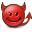)     < set2

>>>>  start(0, 204)   <<<

The devil ment to be the fire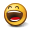I will post a pic or video for all your hard work

6983

Hans

The confusion  im causing is because i was thinking the flames were clockwise on the orginal but ive just uploaded the orginal and the flames go counter clockwise ie starting at 204 around my mirror

6989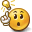once you start working the correct way round// Step 4.  Convert heat to LED colors

for( int j = 1; j < SET_NUM_LEDS; j++) {  //**** here
setPixelHeatColor(j, heat[j] );
setPixelHeatColor(NUM_LEDS – j, heat[j] ); // **** here
}

I dont like asking for help but sometimes its pays

Thank you for your time and instruction because it wouldnt have happen without it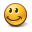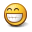6993

Fire effect after Hans gave it a makeover for a infinity mirror

###### Attachments:
6996

Hi Ap0ll0!

Man that looks awesome!!! I love it!!!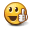Asking for help is never wrong, and it got you started in the right direction, didn’t it?
I’m glad to see that you’ve got it to work – it really looks great!

For those who’d like to see a preview of Ap0ll0’s great effect, see the movie or this preview screenshot …
It looks awesome!

###### Attachments:
Viewing 10 posts - 1 through 10 (of 10 total)

You must be logged in to reply to this topic.